# Distribution of power residues and non-residues

(diff) ← Older revision | Latest revision (diff) | Newer revision → (diff)

The distribution among the numbers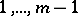of those values offor which the congruence, is solvable (or unsolvable) in integers. Questions on the distribution of power residues and non-residues have been studied most fully in the case modulo a prime number. Let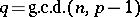. Then the congruence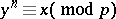is solvable forvalues ofin the set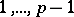and unsolvable for the remainingvalues (see Two-term congruence). However, comparatively little is known about how these values are distributed among the numbers.

The first results about the distribution of power residues were obtained by C.F. Gauss (see ) in 1796. From that time until the work of I.M. Vinogradov only isolated special results were obtained on questions concerning the distribution of power residues and non-residues. In 1915, Vinogradov (see ) proved a series of general results about the distribution of power residues and non-residues, and about primitive roots (cf. Primitive root) moduloamong the numbers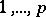. In particular, he obtained the bound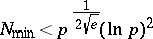for the least quadratic non-residue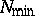, and the bound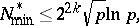whereis the number of distinct prime divisors of, for the least primitive rootmodulo.

In addition, he made a number of conjectures on the distribution of quadratic residues and non-residues (see Vinogradov hypotheses) which stimulated a series of investigations in this area. Thus, Yu.V. Linnik  proved that for sufficiently large, the number of prime numbersin the interval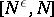for which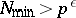does not exceed a certain constant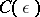, depending only on. Thus, the prime numbersfor which, if they exist at all, are met only very rarely. Another significant step in the work on Vinogradov's conjectures was the theorem of D.A. Burgess : For any fixed sufficiently small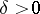, the maximal distance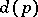between neighbouring quadratic non-residues satisfies the inequality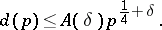In particular, one hasIn these inequalities, the constants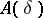,depend only onand not on. The proof of Burgess' theorem is very complicated; it is based on the Hasse–Weil theorem on the number of solutions of the hyper-elliptic congruence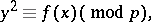the proof of which requires techniques of abstract algebraic geometry. For a simple account of Burgess' theorem see , .

How to Cite This Entry:
Distribution of power residues and non-residues. Encyclopedia of Mathematics. URL: http://encyclopediaofmath.org/index.php?title=Distribution_of_power_residues_and_non-residues&oldid=14183
This article was adapted from an original article by S.A. Stepanov (originator), which appeared in Encyclopedia of Mathematics - ISBN 1402006098. See original article